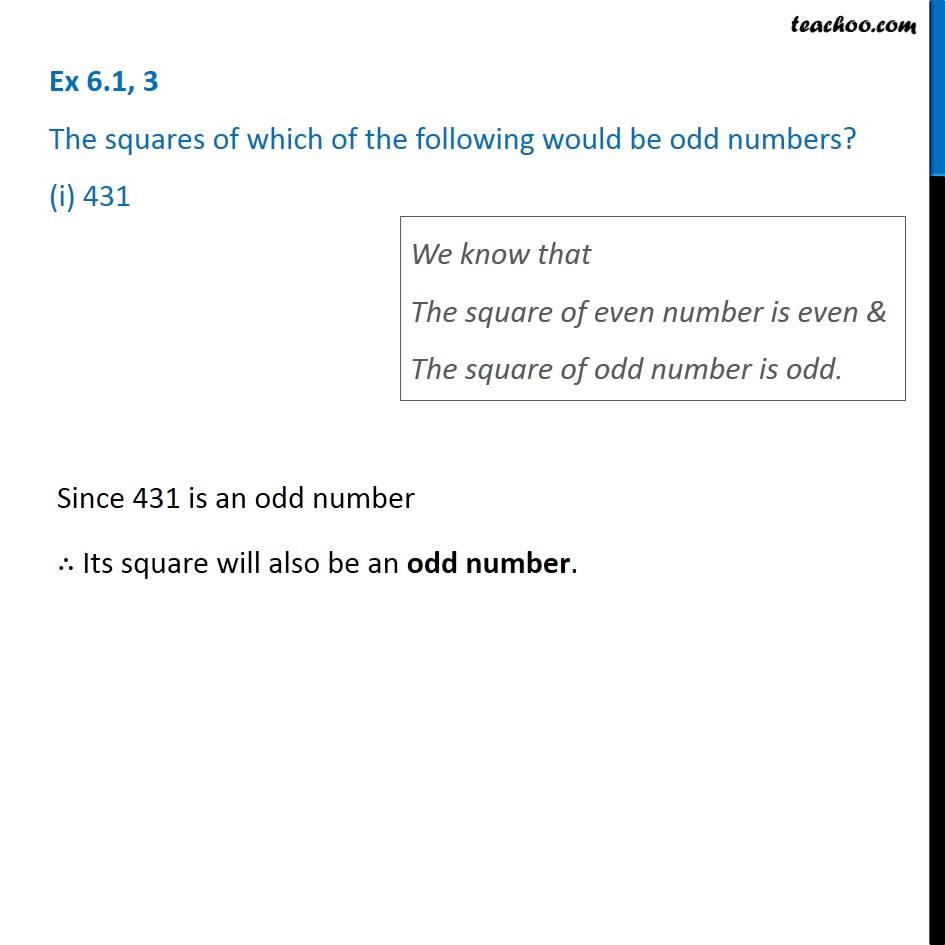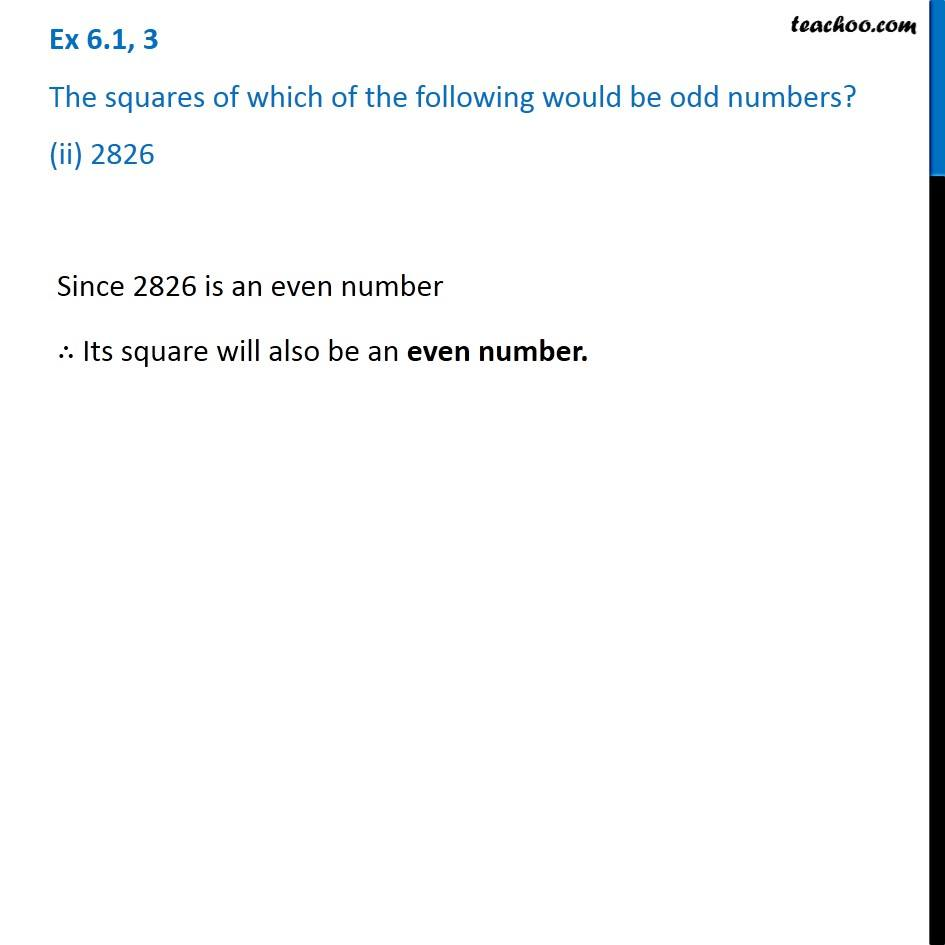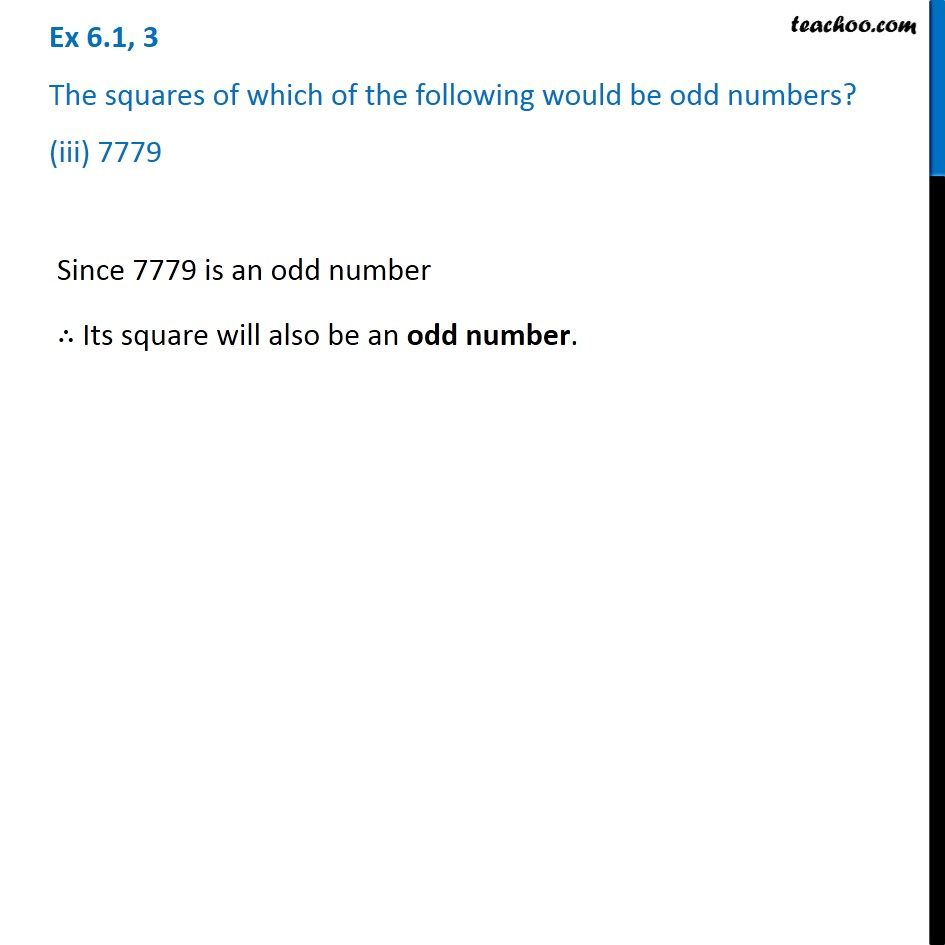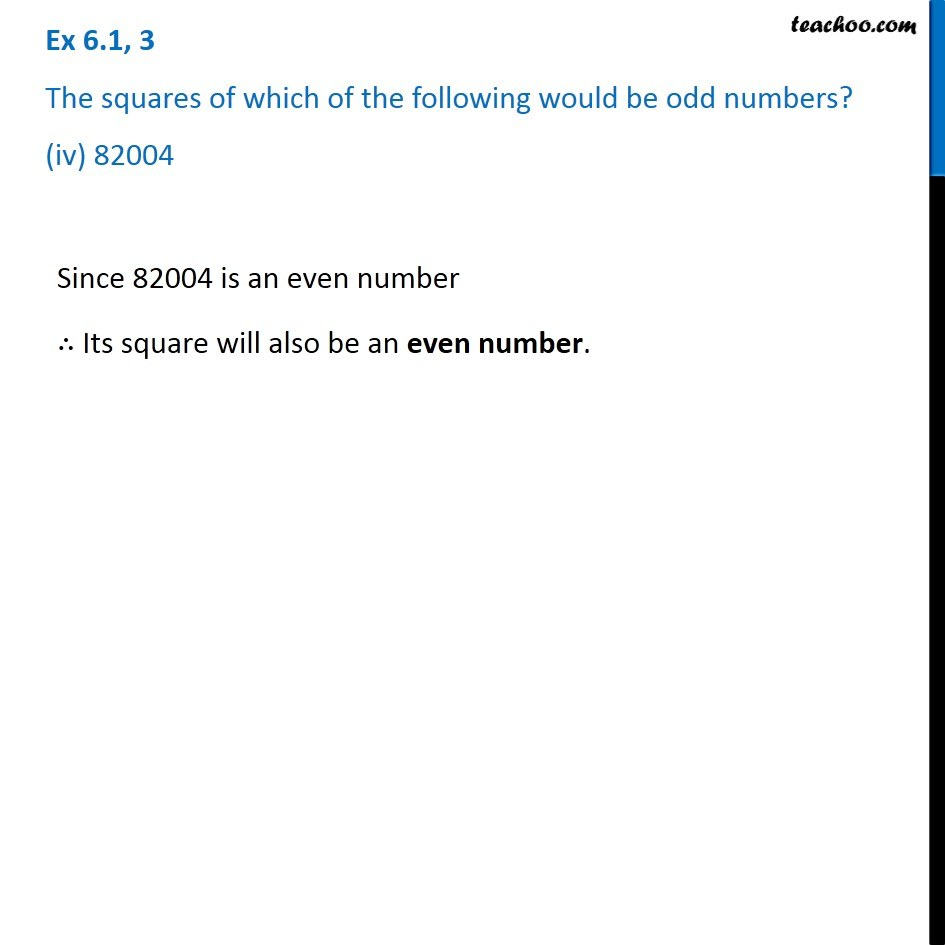Subscribe to our Youtube Channel - https://you.tube/teachoo

1. Chapter 6 Class 8 Squares and Square Roots
2. Serial order wise
3. Ex 6.1

Transcript

Ex 6.1, 3 The squares of which of the following would be odd numbers? (i) 431 We know that The square of even number is even & The square of odd number is odd. Since 431 is an odd number ∴ Its square will also be an odd number. Since 431 is an odd number ∴ Its square will also be an odd number. Ex 6.1, 3 The squares of which of the following would be odd numbers? (ii) 2826Since 2826 is an even number ∴ Its square will also be an even number. Ex 6.1, 3 The squares of which of the following would be odd numbers? (iii) 7779Since 7779 is an odd number ∴ Its square will also be an odd number. Ex 6.1, 3 The squares of which of the following would be odd numbers? (iv) 82004 Since 82004 is an even number ∴ Its square will also be an even number.

Ex 6.1